Browse by Topic
Related Topics

# The Ultimate Univariate Probability Distribution Explorer

In this blog post, we want to report some work in progress that might interest users of probability and statistics and also those who wonder how we add new knowledge every day to Wolfram|Alpha.

Since the beginning in 1988, Mathematica knew not only elementary functions (sqrt, exp, log, etc.) but many special functions of mathematical physics (such as the Bessel function K and the Riemann Zeta function) and number theoretical functions. All together, Mathematica knows now more than 300 such functions. The Wolfram Functions Site lists 300,000+ formulas and identities for these functions. And, based on Mathematica‘s algorithmic computation capabilities and the Functions Site’s identities, most of this knowledge is now easily accessible in Wolfram|Alpha. For example, relation between sin(x) and cos(x), series representations of the Beta function, relation between BesselJ(n, x) and AiryAi(x), differential equation for ellipticF(phi, m), and examples of complicated indefinite integrals containing erf.

But Wolfram|Alpha also knows about many special functions that are not in Mathematica because they are less common or less general. For instance, haversine(x), double factorial binomial(2n, n), Dickman rho(10/3), BesselPolynomialY[6, x], Conway’s base 13 function(4003/371293), and Goldbach function(1000).

Mathematica 7 knew 42 probability distributions; Mathematica 9 knows over 130 (parametric) probability distributions. Based on Mathematica, Wolfram|Alpha can answer a lot of queries about these distributions, such as characteristic function of the hyperbolic distribution or variance of the binomial distribution with p = 1/3, and give general overview pages for queries such as Student’s t distribution or Gumbel distribution.

Similar to special functions, there also exists a whole zoo of probability distributions due to historical reasons and their use in specialized application areas. Some distributions are very specialized, and some are pretty general and have multiple parameters (multiple parameters make a distribution more flexible and versatile, but typically make calculations with them more difficult). In particular, the very specialized ones are not well suited for Mathematica because of their narrow scope. The goal of Wolfram|Alpha is to answer quantitative questions of any form. This, of course, includes probability distributions. This means that in Wolfram|Alpha, which has a broader scope than Mathematica, we do want to support as many probability distributions as possible. It turned out that there are quite a few univariate probability distributions in use. Many of these probability distributions are defined through their probability density function (PDF), which defines the probability of the occurrences of the possible events. But in some application areas it is more natural to start with the inverse cumulative density function (CDF) or a hazard function. Yet, depending on the task at hand, one often needs a PDF, a CDF, various moments, generating functions, entropies, hazard functions, and so on, for a given distribution. And it is often either difficult or even not possible to easily calculate these properties and locate them in the scholarly literature.

So, some time ago, we set out to collect and calculate all that can be collected and calculated about these univariate distributions. We carried out a detailed literature search, and found more than 500 different parametric univariate probability distributions.

There are a few different types of univariate probability distributions. The main classification typically used is discrete versus continuous distributions. One can subdivide these further by their support. Some have finite support, some have half-infinite support, and some are defined over the whole real axis (or at all integers for discrete distributions, respectively). Here is the complete list of all probability distributions that we included in our collection after searching the literature:

Continuous distributions defined on the double infinite interval (-∞, ∞):

Asymmetric Laplace distribution, Bessel alpha distribution, Beta Gumbel distribution, Beta normal distribution, Bramwell Holdsworth Pinton distribution, Burr distribution of the second kind, Burr distribution of the ninth kind, Burr distribution of the sixth kind, Burr distribution of the seventh kind, Burr distribution of the eighth kind, Standard Cauchy distribution, Cauchy distribution, Chotikapanich beta distribution, Dagum distribution of the type 2, Double exponential distribution, Double gamma distribution, Double Weibull distribution, Doubly Pareto uniform distribution, Error distribution, Generalized error distribution, Exponential gamma distribution, Exponential power distribution, Extreme value distribution, Fisher’s z-distribution, Gand distribution, Generalized Cauchy distribution, Generalized Laplacian distribution, Generalized logistic distribution, Generalized logistic distribution of the first kind, Balakrishnan-Leung distribution, Generalized logistic distribution of the third kind, Generalized logistic distribution IV, Generalized logistic distribution of the fourth kind, Generalized McLeish distribution, Gumbel distribution, Gumbel type distribution, Standard Gumbel distribution, Holtsmark distribution, Hyperbolic distribution, Generalized hyperbolic distribution, Hyperbolic secant distribution, Impulse distribution, Johnson SN distribution, Johnson SU distribution, Kotz type distribution, Landau distribution, Laplace double exponential distribution, Linnik distribution, Logistic distribution, Map Airy distribution, McKay Bessel K distribution, McLeish distribution, Meixner distribution, Moyal distribution, Noncentral student t distribution, Standard normal distribution, Normal exponential gamma distribution, Normal inverse Gaussian Lévy distribution, Normal mixture distribution, Pearson distribution of the fourth kind, Pearson distribution of the seven kind, Power normal distribution, Prentice log gamma distribution, Quartic Cauchy distribution, Reflected gamma distribution, Sech distribution, Sech square distribution, Short-tailed symmetric distribution, Sinc square distribution, Skew double exponential distribution, Skew normal distribution, Skew t distribution, Slash distribution, Stable distribution of the zero kind, Standard student t distribution, Student t distribution, Tsallis distribution, Voigt distribution

Continuous distributions defined on half-infinite interval (a, ∞):

Alpha distribution, Amoroso distribution, Arc tangent distribution, Beckmann distribution, Benini distribution, Benktander Gibrat distribution, Benktander Weibull distribution, Beta distribution, Beta exponential distribution, Beta Frechet distribution, Beta Maxwell distribution, Beta modified Weibull distribution, Beta Pareto distribution, Beta prime distribution, Generalized beta prime distribution, Beta Rayleigh distribution, Beta Weibull distribution, Bi exponential distribution, Standard Birnbaum Saunders distribution, Birnbaum Saunders distribution, Birnbaum Saunders type Cauchy distribution, Birnbaum Saunders type Laplace distribution, Birnbaum Saunders type logistic distribution, Birnbaum Saunders type Student t distribution, Bi Weibull distribution, Burr distribution of the third kind, Burr distribution of the tenth kind, Burr distribution of the twelfth kind, Burr type 3 distribution, Chen empirical distribution, Chi distribution, Chi squared distribution, Chotikapanich generalized Pareto distribution, Chotikapanich Ortega distribution, Chotikapanich RGKO distribution, Dagum distribution, Dagum distribution, Dagum type 3 distribution, Davis distribution, Erlang distribution, Exponential distribution, Exponential power distribution, Exponential power I distribution, Exponential power distribution, Exponential Weibull wear out life distribution, Exponentiated exponential distribution, Exponentiated Frechet distribution, Exponentiated Pareto distribution, Exponentiated Weibull distribution, Extended Makeham distribution, Folded Cauchy distribution, Folded normal distribution, Snedecor distribution, Frechet distribution, Gamma distribution, Generalized gamma distribution, Gamma Weibull distribution, Generalized beta distribution, Generalized Birnbaum Saunders distribution, Generalized exponential distribution, Generalized exponential I distribution, Generalized gamma distribution, Generalized logistic distribution of the fifth kind, Generalized Pareto distribution, Geometric extreme exponential distribution, Gibrat distribution, Gompertz distribution, Gompertz Makeham distribution, Half Cauchy distribution, Half logistic distribution, Half normal distribution, Hotteling t squared distribution, Hoyt distribution, Hyper exponential distribution, Hypo exponential distribution, IDB distribution, Increasing decreasing unimodal bathtub shaped distribution, Inverse Burr distribution, Inverse Chi distribution, Inverse chi squared distribution, Scale inverse chi squared distribution, Inverse exponential distribution, Inverse gamma distribution, Generalized inverse gamma distribution, Inverse gaussian distribution, Generalized inverse gaussian distribution, Inverse paralogistic distribution, Inverse Pareto distribution, Inverse Rayleigh distribution, Inverse transformed gamma distribution, Inverted Weibull distribution, Johnson SL distribution, K distribution, Khrgian Mazin distribution, Lévy distribution, Lindley distribution, Logarithm gamma distribution, Logistic exponential distribution, Log Laplace distribution, Log Laplace 1 distribution, Log-logistic distribution, Lognormal distribution, Log skew normal distribution, Log skew t distribution, Lomax distribution, Maccone distribution, Makeham distribution, Maxwell distribution, Max stable distribution, McKay Bessel distribution, Mielke beta kappa distribution, Mittag Leffler distribution, Modified Weibull distribution, Muth distribution, Nakagami distribution, Noncentral Chi distribution, Noncentral Chi squared distribution, Noncentral f ratio distribution, Paralogistic distribution, Pareto distribution, Pareto distribution of the fourth kind, Lomax distribution, Pearson distribution of the fifth kind, Pearson distribution of the sixth kind, Generalized Pearson distribution of the third kind, Generalized Pearson distribution of the fifth kind, Generalized Pearson distribution of the sixth kind, Positive normal distribution, Powerlog normal distribution, Pseudo Weibull distribution, q exponential B distribution, q Gamma B distribution, q Weibull B distribution, Rayleigh distribution, Rayleigh distribution, Reciprocal inverse Gaussian distribution, Rice distribution, Rice distribution, Scaled Chi distribution, Scaled Chi square distribution, Scaled inverse Chi distribution, Scaled inverse Chi square distribution, Singh Maddala distribution, Stacy distribution, Standard gamma distribution, Stoppa distribution of the first kind, Stretched exp distribution, Suzuki distribution, Wakeby distribution, Wald distribution, Weibull distribution, Weibull type distribution, Wilson Hilferty distribution

Continuous distributions defined on a finite interval (a, b):

Anglit distribution, Arc-Sine distribution, Bates distribution, Beta distribution, Bradford distribution, Burr distribution of the first kind, Burr distribution of the fourth kind, Burr distribution of the fifth kind, Burr distribution of the eleventh kind, Cardioid distribution, Chotikapanich distribution, Cosine distribution, Curve fitting BET sigmoidal distribution, Curve fitting box Lucas distribution, Curve fitting Chapman model distribution, Curve fitting exp distribution, Curve fitting exponential distribution, Curve fitting exponential growth distribution, Curve fitting exponential root fit distribution, Curve fitting Freundlich model distribution, Curve fitting Gaussian distribution, Curve fitting Hill model distribution, Curve fitting Hoerl model distribution, Curve fitting hyperbola distribution, Curve fitting hyperbolic cosine distribution, Curve fitting hyperbolic secant distribution, Curve fitting inverse hyperbola distribution, Curve fitting Langmuir distribution, Curve fitting linear distribution, Curve fitting logarithmic distribution, Curve fitting logarithmic exponential distribution, Curve fitting logistic model distribution, Curve fitting modified cosine distribution, Curve fitting modified gamma distribution, Curve fitting modified geometric distribution, Curve fitting modified power distribution, Curve fitting power distribution, Curve fitting pursuit curve distribution, Curve fitting quadratic distribution, Curve fitting reciprocal power distribution, Curve fitting reciprocal quadratic distribution, Curve fitting reciprocal sine distribution, Curve fitting shifted power distribution, Curve fitting Stirling distribution, Curve fitting wave form distribution, Double Log distribution, Generalized extreme value distribution, FMKL generalized Tukey lambda distribution, Generalized beta distribution, Beta distribution, Generalized half logistic distribution, Generalized normal distribution, Generalized Topp Leone distribution, Generalized trapezoidal distribution, Geometric stable distribution, Half Halo distribution, Hyperbolic secant distribution, Johnson SB distribution, Kumaraswamy distribution, Leipnik distribution, Logarithm gamma distribution, Log beta distribution, Logit normal distribution, Max stable distribution, McCullagh distribution, Mini max distribution, Min stable distribution, Noncentral beta distribution, Nukiyama Tanasawa distribution, Ogive distribution, Pareto distribution, Pearson distribution of the first kind, Pearson distribution of the second kind, Pearson distribution of the third kind, Generalized Pearson distribution of the first kind, PERT distribution, Standard power distribution, Power distribution, q exponential distribution, q gamma distribution, q Weibull distribution, Reciprocal distribution, Reflected generalized Topp Leone distribution, Reflected power distribution, Reflected Topp Leone distribution, RS generalized Tukey lambda distribution, Shifted log logistic distribution, Slope distribution, Stable distribution of the first kind, Topp Leone distribution, Trapezoidal distribution, Standard triangular distribution, Triangular statistical distribution, Truncated exponential distribution, Truncated normal distribution, Two parameter beta distribution, Two sided Ogive distribution, Two sided power distribution, TSP distribution, Two sided Slope distribution, Uneven two sided power distribution, Uniform distribution, Uniform sum distribution, Upper truncated Pareto distribution, u quadratic distribution, von Mises distribution, Wigner semi circle distribution, Wrapped up Cauchy distribution

Discrete distributions defined on the double infinite interval (-∞, ∞):

Skellam distribution

Discrete distributions defined on half-infinite interval [a, ∞):

Beta geometric distribution, Beta binomial distribution, Binomial-negative binomial distribution, Binomial Poisson distribution, Borel distribution, Borel Tanner distribution, Consul distribution, Conway-Maxwell-Poisson distribution, Delta Consul distribution, Delta Felix distribution, Delta Geeta distribution, Delta Katz distribution, Delta negative binomial distribution, Delta Otter distribution, Delta Poisson distribution, Delta Sunil distribution, Delta Teja distribution, Delta Ved distribution, Discrete Weibull distribution, Generalized binomial distribution, Engset distribution, Extended negative binomial distribution, Felix distribution, Geeta distribution, Generalized Katz distribution, Generalized logarithmic series distribution, Generalized negative binomial distribution, Generalized power series distribution, Geometric distribution, Haight distribution, Haight zeta distribution, Hermite distribution, Holla distribution, Inverse binomial distribution, Katz distribution, Lagrange-Poisson distribution, Lagrangian distribution of the first kind, Lagrangian distribution of the second kind, Logarithmic-negative binomial distribution, Logarithmic series distribution, Lost games distribution, Menzerath Altmann distribution, Modified Felix distribution, Modified Ved distribution, Negative binomial binomial distribution, Negative binomial distribution, Negative binomial Poisson distribution, Otter distribution, Pascal distribution, Poisson binomial distribution, Poisson Consul distribution, Poisson distribution, Poisson gamma distribution, Poisson LS distribution, Poisson-negative binomial distribution, Polya Aeppli distribution, Rectangular binomial distribution, Rectangular negative binomial distribution, Rectangular Poisson distribution, Shenton distribution, Sunil distribution, Teja distribution, Ved distribution, Waiting-time negative binomial distribution, Waring distribution, Waring Yule distribution, Yule-Simon distribution, Zero-inflated Poisson distribution, Zeta distribution, Zipf distribution

Discrete distributions defined on a finite interval [a, b]:

Benford distribution, Bernoulli distribution, Beta binomial distribution, Binomial distribution, Bose Einstein distribution, Classical matching distribution, Coin tossing distribution, Deformed Katz-Powell distribution, Degenerate distribution, Discrete uniform distribution, Fisher noncentral hypergeometric distribution, Hypergeometric distribution, Laplace Haag matching distribution, Naor distribution, Negative hypergeometric distribution, Polya distribution, Quasi binomial distribution of the first kind, Rademacher distribution, Riff Shuffle distribution, Specified occupancy distribution, Wallenius distribution, Zipf distribution, Zipf-Mandelbrot distribution

Various of these distributions occur with a varying number of parameters, adding another few dozen distributions to this list. Some of these above distributions, such as the Bernoulli and Poisson distributions, are well known and some are not so well known, but a quick web search points to some papers about them (e.g. the McCullagh distribution or the Wallenius distribution).

Mathematically, univariate probability distributions are just univariate functions with some supplementary conditions, such as having a monotonic increasing CDF and normalizability. In this sense they are really quite similar to the special functions of mathematical physics. Although univariate probability distributions are in most cases having a PDF made from elementary functions, their CDF and other properties quite frequently contain higher special functions. To avoid propagating potential typos and mistakes and not depend on certain conventions, we just extracted the defining properties (mostly the PDF) of the distributions from the literature and derived all relevant properties from scratch, carefully taking into account convergence conditions for integrals and sums. Calculating properties such as moments, generating functions, and entropies results often in quite challenging integrals and sums. As much as possible, we expressed these through named mathematical functions.

Concretely, the properties we calculated (on the base of the defining formulas) for each probability distribution were:

PDF (probability density function), CDF (cumulative density function), support, parameter conditions (conditions on the parameters such that the resulting function is a distribution), mean, variance, standard deviation, skewness, kurtosis, median, quantile, quartiles, mode, characteristic function, moments, central moments, factorial moments, ascending factorial moment, cumulants, factorial cumulant, ascending factorial cumulant, moment ratio, root mean square, moment generating function, central moment generating function, factorial moment generating function, descending factorial moment generating function, ascending factorial moment generating function, cumulant generating function, factorial cumulant generating function, descending factorial cumulant generating function, ascending factorial cumulant generating function, geometric mean, harmonic mean, Gini mean, inverse CDF, inverse survival function, sparsity, interquartile range, quartile deviation, quartile skewness, differential equation for the PDF, differential equation for quantile, Shannon entropy, Renyi entropy, Tsallis entropy, Landsberg Vedral entropy, Abe entropy, Kaniadakis entropy, Sharma Mittal entropy, Gini index, survival function, cumulative hazard function, hazard function, Lorenz curves, mean residual life function, PDF visualizations, CDF visualizations

These are about 60 properties. Multiplied by 500 probability distributions, this resulted in more than 30,000 formulas; 31,591, to be precise. (Not every property applies to every distribution; for example, the Cauchy distribution does not have higher moments due to its fat tail, and some properties cannot be expressed in closed form through named mathematical functions.) A typical mathematics handbook has at most 20 to 25 formulas per page (e.g. integrals that are displayed as multiline objects spanning more than a single line). This means that putting all these formulas into a book would easily give a 1,200-page book (even without including any of the plots of the distributions). A good chunk of mathematical knowledge—just for one of the many ongoing additions to Wolfram|Alpha.

Incorporating these probability distributions and their properties into Wolfram|Alpha will happen in the near future. To give you an idea about the scope and depth of coverage, we thought it might be interesting for Wolfram|Alpha users to have a preview of these formulas and our team’s work to strive for a comprehensive coverage. So, how to present such a large amount of formulas in a comprehensible way? We decided on an interactive “single-page” version of the complete collection, in the form of a result of Mathematica‘s Manipulate function, similar to the format of the interactive documents at the Wolfram Demonstrations Project. This allow us to put all the 30,000+ formulas in a uniform, easily browsable format, and all in initially about 800×900 pixels (less than 1 megapixel). The file can be downloaded here as a Computable Document Format (CDF) file. A quite similar version of this interactive viewer has been used in the development phase of the project. Because this interactive document allows you to explore the properties of univariate probability distributions, and everything is implemented in Mathematica, we call it “The Ultimate Univariate Probability Distribution Explorer.”

Here is what the .cdf document looks like after opening. (We choose the well-known normal distribution and its most important characteristics as the default.)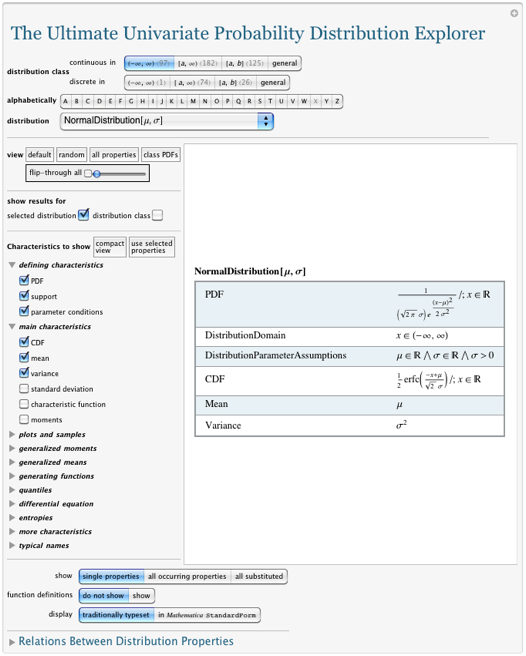On the top are the menus to choose the type of distribution and the concrete distribution, and on the left are the checkboxes to specify which properties to display. The initial state shows the well-known properties of the normal distribution. The pulldown menu on the top also allows you to choose the function alphabetically.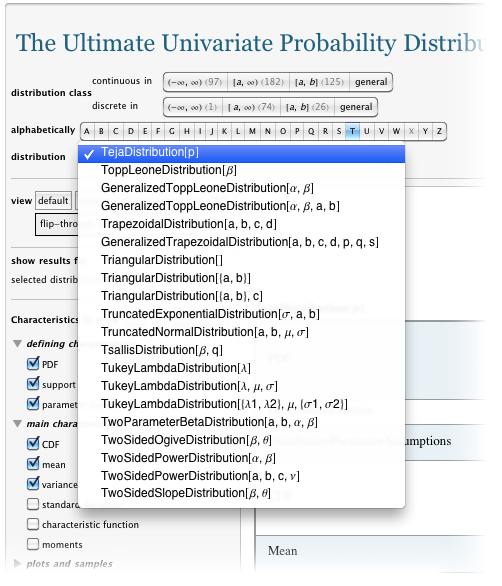Mousing over one of the distribution classes, for instance over the continuous distributions over a half-infinite interval, shows the list of all distributions from that class.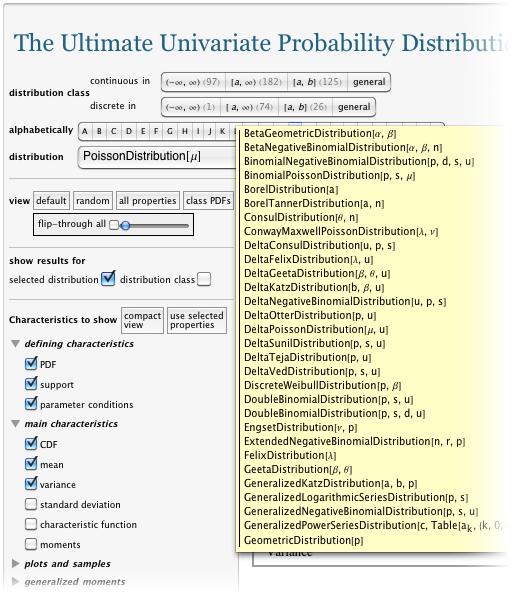To get some idea of what is included, just press the “random” button. It will randomly select a distribution and some properties. As mentioned, properties are often integrals and sums containing the distribution. And some of these integrals and sums can be quite complicated. As much as possible, the Ultimate Univariate Probability Distribution Explorer returns a closed form for these integrals and sums. Here is a state reached from using the random button that shows this quite clearly (because of the size of the result, we show only part of it).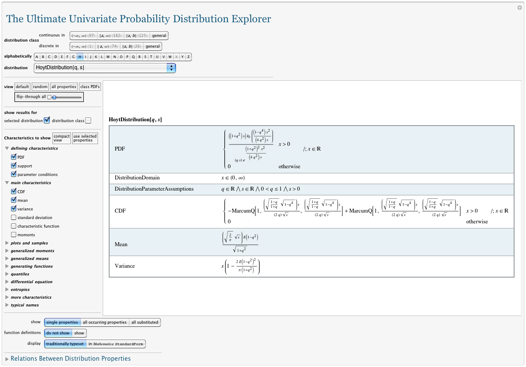There is also an “all properties” button. Use it with care so you aren’t scared about how complicated certain properties of even simple distributions can be. Here are some entropies for the lognormal distribution.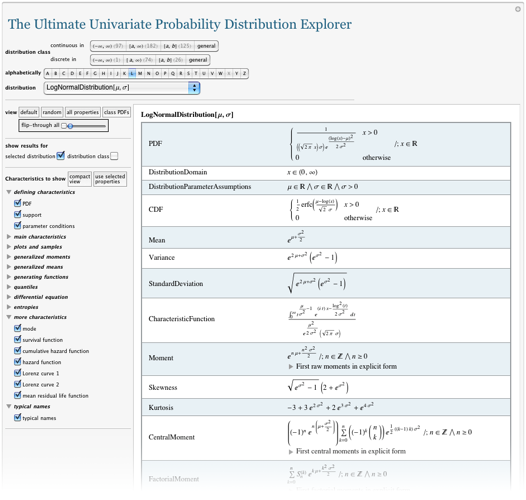One can also compare the PDFs (and CDFs etc.) for a whole class of distributions. For instance, here is part of the result that compares the PDFs for all discrete distributions defined on a finite interval.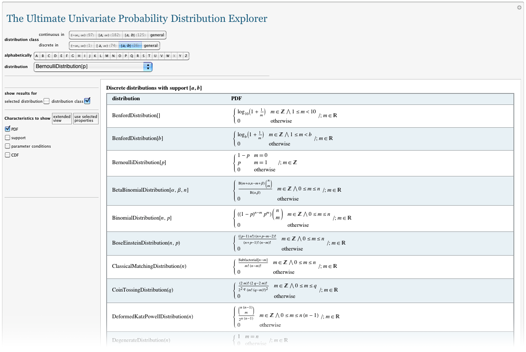Or, for quickly flipping through a lot of pages with the same information, you can use the “flip-through” mode and move the corresponding slider.

In addition to looking up formulas, the Explorer also allows you to view plots of the PDF and CDF (and their derivatives). And the left-hand side in the property specification selects the relevant plots. Here are some plots of the (generalized) Student’s t distribution.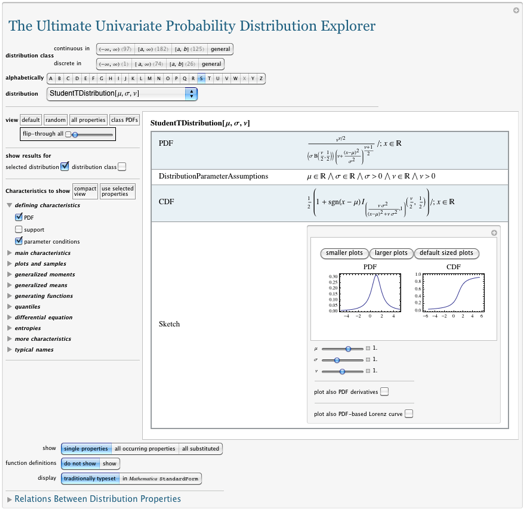The Univariate Probability Explorer has a variety of other features, such as generating sample random numbers for a given distribution; the possibility to display all results in Mathematica StandardForm so you can easily copy and paste them and carry out computations with the formulas; a list of often-used names for a distribution, including definitions of special functions used in results (such as hypergeometric functions, Beta functions, etc.); and more. The next screen shot shows various generalized moments for the Poisson distribution together with some links for the occurring special functions.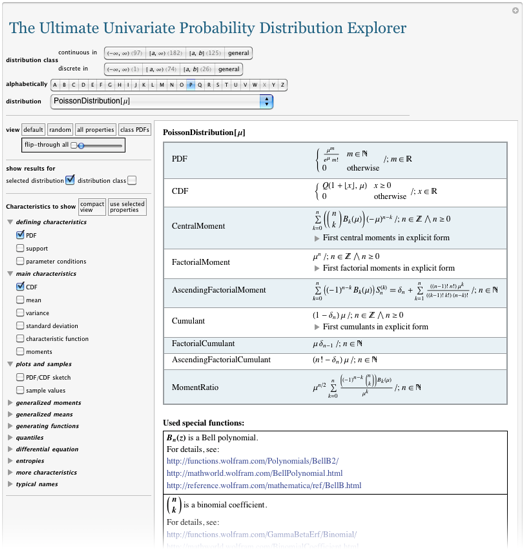Instead of selecting a concrete probability distribution, you can also select a general (abstract) distribution (using the two right-most “general “buttons) in the “distribution class” menu. In this case, the defining formulas for the selected properties are shown. Here are some selected definitions for a general discrete distribution.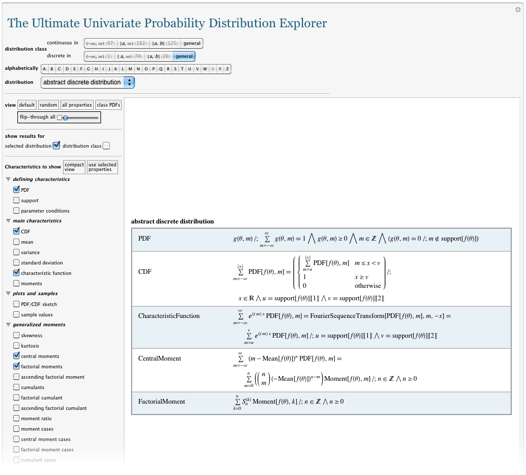Before coming to the end, we want to mention the “Relations between distribution properties” feature at the bottom of the Explorer. This section serves as a handy set of definitions and connection formulas. These identities follow from the definitions of the properties and hold for any distribution. For instance, for a given CDF of a continuous probability distribution, how do you get the PDF?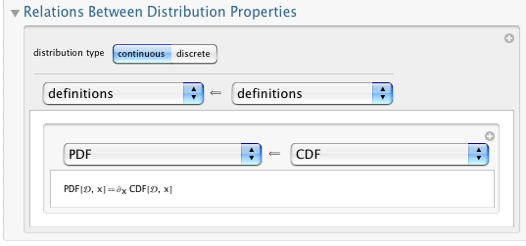(This section adds another 2,500 formulas to the Explorer, which were not included in the above count.) And here is a more complicated example: Given the sparsity, how do you calculate the variance?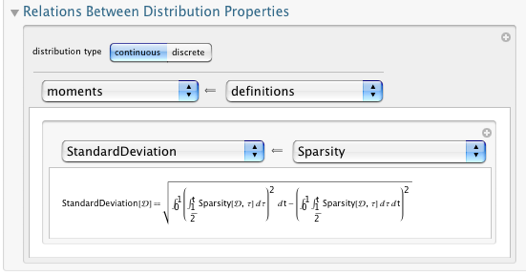This ends our quick overview of the features and identities of the Ultimate Univariate Probability Distribution Explorer—a small, but useful side effect of a formula-collecting initiative for Wolfram|Alpha. In addition to giving users some idea of how we assemble mathematical information in Wolfram|Alpha, we hope readers will find it a handy reference for their work with probability distributions, or a rich source for potential exercises in integration and probability theory homeworks.

Now that we have assembled the data for univariate probability distributions, we will start adding these distributions and their properties to Wolfram|Alpha to make them even easier to use than through the interactive CDF document described here. In Wolfram|Alpha these distributions will become accessible through the natural language interface of Wolfram|Alpha. In the coming months, Wolfram|Alpha will know about many of the distributions. Once again, you can download the Univariate Probability Explorer here.